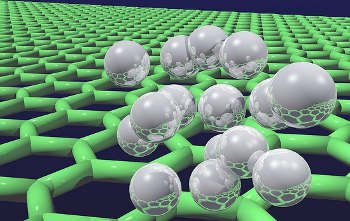# How often do quantum systems violate the second law of thermodynamics?

The likelihood of seeing quantum systems violating the second law of thermodynamics has been calculated by UCL scientists.

In two papers, published in Physical Review X and funded by the Engineering and Physical Sciences Research Council, the team determined a more precise version of a basic law of physics – which says that disorder tends to increase with time unless acted on by an outside force – and applied it to the smallest quantum systems.Quantum Hydrogen on Graphene (Image credit: UCL Mathematical & Physical Sciences)

The vast majority of the time, the second law of thermodynamics is obeyed. It says that a cup of hot coffee in a cold room will cool down rather than heat up, and a collection of coins all initially heads up will likely produce a mixture of heads and tails when given a shake. In fact, it is thanks to the second law of thermodynamics that we instantly recognise when we are watching a movie backwards,” explained PhD student Alvaro M. Alhambra (UCL Physics & Astronomy).

The team say that situations which break the second law of thermodynamics are not ruled out in principle, but are rare.

We wanted to find out by how much disorder increases, and if disorder sometimes decrease with some probability. These questions become important for small quantum systems where violations of the second law can happen with a significant probability,” added co-author Professor Jonathan Oppenheim (UCL Physics & Astronomy).

The team, which also included Dr Christopher Perry (previously at UCL and now a researcher at the University of Copenhagen), revealed how the second law of thermodynamics functions when applied to the smallest scales of the microscopic world and the calculated the maximum probability of observing a violation.

Dr Lluis Masanes (UCL Physics & Astronomy), said: “The probability of the law being violated is virtually zero for large objects like cups of tea, but for small quantum objects, it can play a significant role. We wanted to determine the probability of violations occurring, and wanted to prove a more precise version of the second law of thermodynamics.”

The second law is usually expressed as an inequality e.g., the amount of energy flowing from the cup to the air has to be larger than zero. However, it can also be expressed as an equality instead, saying precisely how much energy flows from the air to the cup and with what probabilities. This equality version of the second law can be proven for the most general process allowed by the laws of quantum mechanics.

In addition, this new formulation of the second law contains a very large amount of information, dramatically constraining the probability and size of fluctuations of work and heat and, tells us that the particular fluctuations that break the second law only occur with exponentially low probability.

These findings are critical to nanoscale devices, and the rapidly developing field of quantum technologies.

*Source: University College London

(Visited 19 times, 1 visits today)